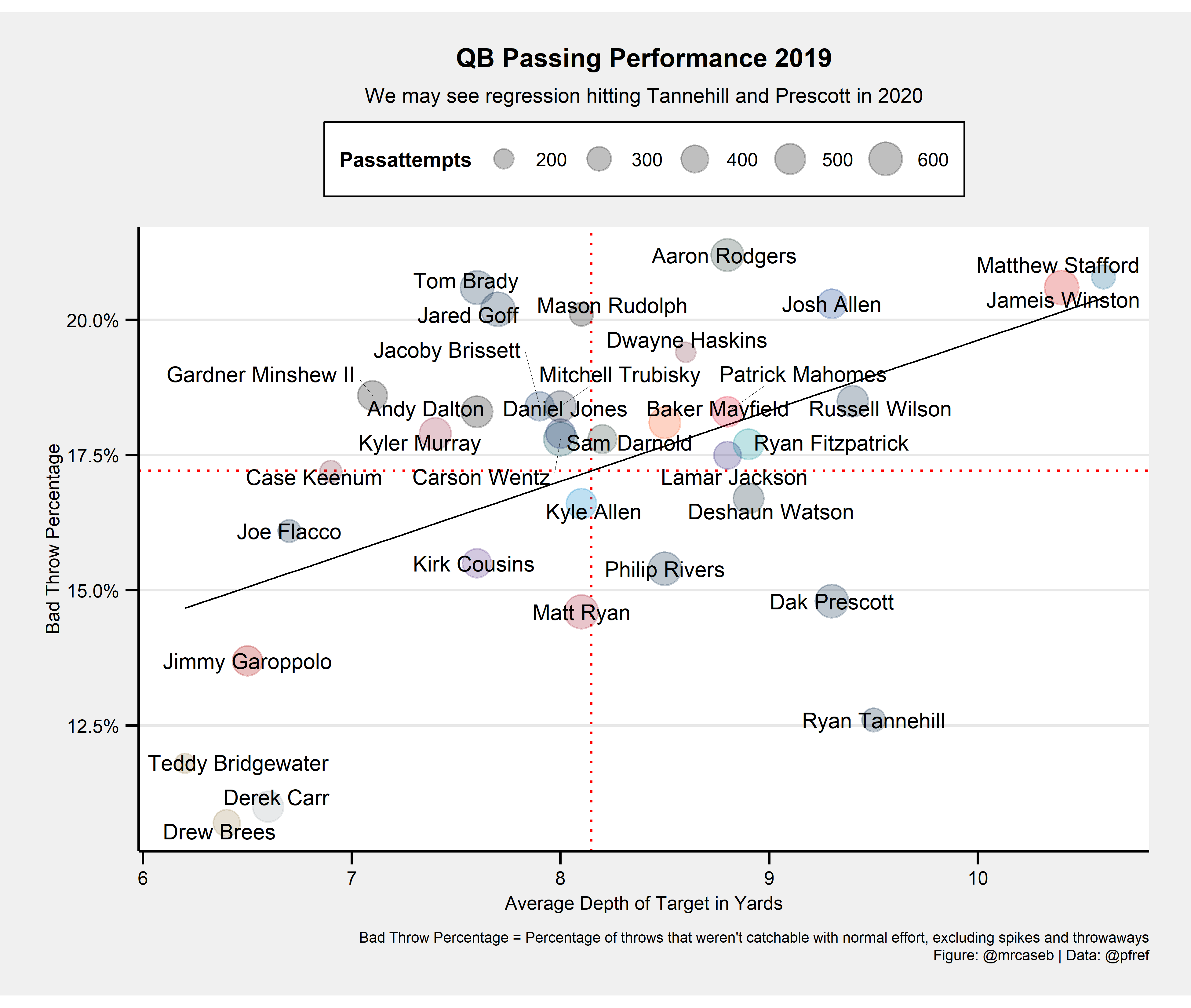PFR’s Bad Throw Percentage for Quarterbacks

This article shows how to scrape football data from Pro Football Reference and how to plot the bad throw percentage data for quarterbacks.

08-18-2020

Preface

One of the most important sources for football related data is Pro Football Reference (short PFR). There are a ton of useful stats and I would like to look into their “Bad Throw Percentage” defined as

Percentage of poor throws per pass attempt, excluding spikes and throwaways

PFR provides it’s data on static html websites which makes it easy to scrape them. This will be demonstrated below.

Get the data and save it locally

Scraping data means traffic for the provider. We don’t want to annoy them so we scrape them once and save them locally. This can be done with the following code (please note it saves only the for this example relevant variables and you might want to change that):

library(tidyverse)
library(rvest)

# scrape data from PFR----------------------------------------------------------
pfr_raw <- url %>%
html_table() %>%
as.data.frame()

# clean the scraped data--------------------------------------------------------

# rename the columns as the actual column names are saved in the first row now
colnames(pfr_raw) <- make.names(pfr_raw[1, ], unique = TRUE, allow_ = TRUE)

# drop the first row and select the columns we are interested in
pfr <- pfr_raw %>%
slice(-1) %>%
select(Player, Tm, IAY.PA, Bad., Att) %>%
rename(team = Tm) %>%
mutate(
# pfr uses different team abbreviations than nflfastR, fix them
team = case_when(
team == "GNB" ~ "GB",
team == "KAN" ~ "KC",
team == "NOR" ~ "NO",
team == "NWE" ~ "NE",
team == "SFO" ~ "SF",
team == "TAM" ~ "TB",
TRUE ~ team
),
# repair player names
Player = str_replace(Player, "\\*", ""),
Player = str_replace(Player, "\\+", ""),

# make interesting columns numeric
IAY.PA = as.numeric(IAY.PA),
Passattempts = as.numeric(Att)
) %>%
# join colors and logos from nflfastR
left_join(nflfastR::teams_colors_logos, by = c("team" = "team_abbr"))

# save to disk------------------------------------------------------------------
# binary

# ASCII

Create the plot

The data we want to have a look at is now saved locally and can be used to create the plot:

library(tidyverse)

chart_data %>%
ggplot(aes(x = IAY.PA, y = Bad. / 100)) +
geom_hline(aes(yintercept = mean(Bad. / 100)), color = "red", linetype = "dotted") +
geom_vline(aes(xintercept = mean(IAY.PA)), color = "red", linetype = "dotted") +
geom_smooth(method = "lm", se = FALSE, color = "black", size = 0.3) +
geom_point(color = chart_data\$team_color, aes(cex = Passattempts), alpha = 1 / 4) +
ggrepel::geom_text_repel(aes(label = Player), force = 1, point.padding = 0, segment.size = 0.1, size = 3) +
scale_y_continuous(labels = scales::percent) +
scale_size_area(max_size = 6) +
labs(
x = "Average Depth of Target in Yards",
caption = "Bad Throw Percentage = Percentage of throws that weren't catchable with normal effort, excluding spikes and throwaways\nFigure: @mrcaseb | Data: @pfref",
title = "QB Passing Performance 2019",
subtitle = "We may see regression hitting Tannehill and Prescott in 2020"
) +
ggthemes::theme_stata(scheme = "sj", base_size = 8) +
theme(
plot.title = element_text(face = "bold"),
plot.caption = element_text(hjust = 1),
axis.text.y = element_text(angle = 0, vjust = 0.5),
legend.title = element_text(size = 8, hjust = 0, vjust = 0.5, face = "bold"),
legend.position = "top",
aspect.ratio = 1 / 1.618
) +
NULLCorrections

If you see mistakes or want to suggest changes, please create an issue on the source repository.

Reuse

Text and figures are licensed under Creative Commons Attribution CC BY-NC 4.0. Source code is available at https://github.com/mrcaseb/open-source-football, unless otherwise noted. The figures that have been reused from other sources don't fall under this license and can be recognized by a note in their caption: "Figure from ...".